# Nth lowest or nth highest value in Excel

In this article, we will learn how to get Nth lowest or nth highest value in Excel.

In simple words, while working with data numbers, sometimes we need to find the second or third highest number from the array. Or you need to find out the 10th lowest from the bottom of the array You can perform the solution to this problem easily using the excel functions as explained below.

Nth lowest from the array

How to solve the problem?

For this article we will be required to use the LARGE function. Now we will make a formula out of the function. Here we are given a range and we need to nth values from the top in given range.

Generic formula:

= LARGE ( range, n )

range : range of values

n : nth lowest from the range
Example:

All of these might be confusing to understand. So, let's test this formula via running it on the example shown below.

Here we have a range of values from A2:A18 and we need to find the nth largest value from the given array.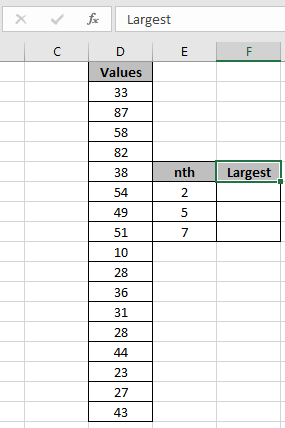Here the range is given as using the named range excel tool.

Firstly, we need to find the 2nd highest from the array. Values named range for the array A2:A18.
Use the Formula:

= LARGE ( Values, E7 )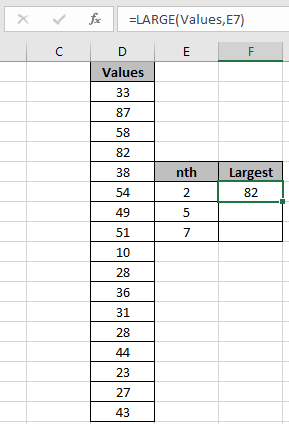As you can see in the above snapshot the 2nd lowest value from the array is 82.

Now get the 5th highest and 7th highest value by changing the value of n in the formula.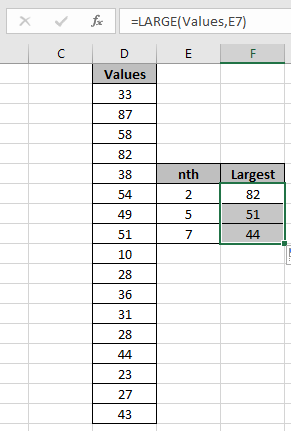As you can see the formula works fine it gets all the value using Excel LARGE function.

Nth lowest from the array

How to solve the problem?

For this article we will be required to use the SMALL function. Now we will make a formula out of the function. Here we are given a range and we need to nth values from the bottom in given range.

Generic formula:

= SMALL ( range, n )

range : range of values

n : nth lowest from the range

Example:

All of these might be confusing to understand. So, let's test this formula via running it on the example shown below.

Here we have a range of values from A2:A18 and we need to find the nth smallest value from the given array.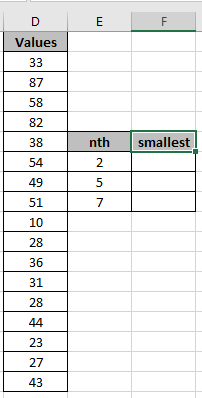Here the range is given as using the named range excel tool.

Firstly, we need to find the 2nd lowest from the array.
Values named range for the array A2:A18.
Use the Formula:

= SMALL ( Values, E7 )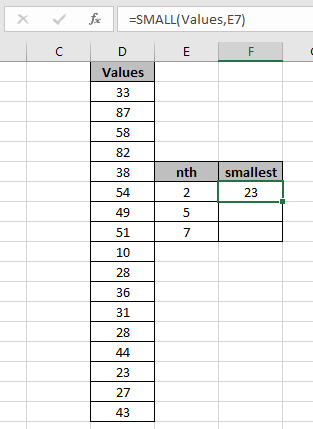As you can see in the above snapshot the 2nd lowest value from the array is 23

Now get the 5th lowest and 7th lowest value by changing the value of n in the formula.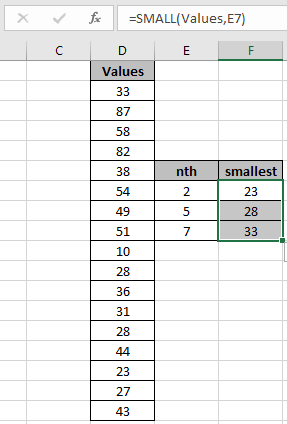As you can see the formula works fine it gets all the value using Excel SMALL function.

Here are some observational notes shown below.

Notes:

1. The formula only works with numbers.
2. Value of n cannot be less than 1 or greater than length of array or else it return error

Hope this article about how to Return Nth lowest or nth highest value in Excel is explanatory. Find more articles on SUMPRODUCT functions here. Please share your query below in the comment box. We will assist you.

Related Articles

How to use the SUMPRODUCT function in Excel: Returns the SUM after multiplication of values in multiple arrays in excel.

SUM if date is between : Returns the SUM of values between given dates or period in excel.

Sum if date is greater than given date: Returns the SUM of values after the given date or period in excel.

2 Ways to Sum by Month in Excel: Returns the SUM of values within a given specific month in excel.

How to Sum Multiple Columns with Condition: Returns the SUM of values across multiple columns having condition in excel

How to use wildcards in excel : Count cells matching phrases using the wildcards in excel

Popular Articles

50 Excel Shortcut to Increase Your Productivity

Edit a dropdown list

Absolute reference in Excel

If with conditional formatting

If with wildcards

Vlookup by date

Convert Inches To Feet and Inches in Excel 2016

Join first and last name in excel

Count cells which match either A or B

Terms and Conditions of use

The applications/code on this site are distributed as is and without warranties or liability. In no event shall the owner of the copyrights, or the authors of the applications/code be liable for any loss of profit, any problems or any damage resulting from the use or evaluation of the applications/code.